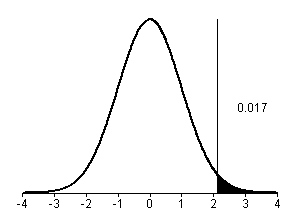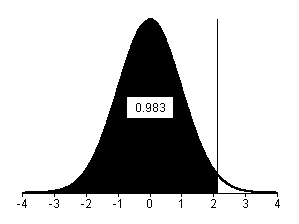# One tailed p-values

## 2004-04-12

Dear Professor Mean, SPSS produces two-tailed p-values, but I want a one-tailed p-value. How do I get this?

First, let’s review what the difference between a one-tailed and two-tailed p-value is.

A two sided hypothesis states that there is a difference between the treatment/exposure group and the control group, but does not specify in advance what direction you think this difference will be. A one sided hypothesis states a specific direction (e.g., increase).

If you expect that a change in either direction is possible and that changes in either direction are interesting, then you should use a two sided hypothesis.

If changes in one direction are uninteresting and unpublishable, then use a one sided hypothesis. Also if a change in the unexpected direction is equivalent in practice to no change, then use a one sided hypothesis.

The best example of this is when you are comparing a new therapy to an existing therapy, where the new therapy is much more expensive, your only concern is to show that the new therapy is better. If it turns out that then new therapy is equal to or worse than the standard therapy, you will not adopt it.

A two-tailed p-value is a p-value computed for a two-tailed hypothesis. A one-tailed p-value is computed for a one-tailed hypothesis.

To compute a one-tailed p-value, just take the two-tailed p-value and cut it in half. Just make sure that the effect is going in the direction you hypothesized. If you believe that an intervention can only have a positive impact and your two-sided p-value is 0.034, then a one-sided p-value would be 0.017, assuming that the treatment behaved as you expected it to. But if the effect were unexpectedly negative, your p-value would be 0.983 and not 0.017.The graph shown above illustrates the calculation of a two-sided p-value. The p-value is the sum of the positive and negative tails of the distribution, which adds up to 0.034.This graph shows how the p-value changes to a one-sided p-value with the change going in the direction that you hypothesized. In this case, the p-value is half of the two-sided p-value or 0.017.This graph shows how the p-value changes with the change going in the opposite direction from what you hypothesized. In this case, the p-value is 1-p/2 or 0.983.

You can find an earlier version of this page on my original website.